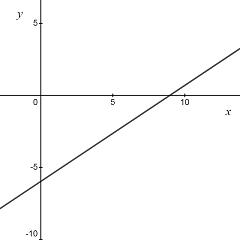# Sketch the graph of 2x-3y=18. What are the intercepts and the slope of a line?

## Question:

Sketch the graph of {eq}2x-3y=18 {/eq}. What are the intercepts and the slope of a line?

## Line Equation:

A line in the x-y plane can be expressed in the explicit form

{eq}y = mx + x_0 {/eq}

where m is the slope (angular coefficient) and {eq}x_0 {/eq} is the intercept.

Given the line equation

{eq}2x-3y=18 {/eq}

we rewrite it in explicit form

{eq}\displaystyle 2x-3y=18 \Rightarrow y=\frac{2}{3}x-6. {/eq}

The x-intercept of the line is

{eq}\displaystyle y= 0 \Rightarrow x=9 {/eq}

and the y-intercept is

{eq}\displaystyle x= 0 \Rightarrow y=-6. {/eq}

The slope of the line is

{eq}\displaystyle m=\frac{2}{3}. {/eq}

A sketch of the line is reported in the figure below.